# NCERT Solutions class-11 Maths Exercise 14.2## myCBSEguide App

CBSE, NCERT, JEE Main, NEET-UG, NDA, Exam Papers, Question Bank, NCERT Solutions, Exemplars, Revision Notes, Free Videos, MCQ Tests & more.

Exercise 14.2

1. Write the negation of the following statements:

(i) Chennai is the capital of Tamilnadu.

(ii)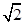is not a complex number.

(iii) All triangles are not equilateral triangle.

(iv) The number 2 is greater than 7.

(v) Every natural number is an integer.

Ans. (i) Negation of statement is: Chennai is not the capital of Tamilnadu.

(ii) Negation of statement is:is a complex number.

(iii) Negation of statement is: All triangles are equilateral triangle.

(iv) Negation of statement is: The number 2 is not greater than 7.

(v) Negation of statement is: Every natural number is not an integer.

2. Are the following pairs of statements negations of each other:

(i) The number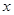is not a rational number.

The numberis not an irrational number

(ii) The numberis a rational number.

The numberis an irrational number

Ans. (i) Let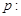The numberis not a rational number.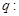The numberis not an irrational number.

Now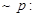The numberis a rational number.

And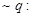The numberis an irrational number.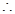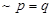and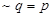Therefore,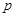and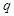are negation of each other.

(ii) LetThe numberis a rational number.The numberis an irrational number.

NowThe numberis not a rational number.

AndThe numberis not an irrational number.andTherefore,andare negation of each other.

3. Find the component statements of the following compound statements and check whether they are true or false:

(i) Number 3 is prime or it is odd.

(ii) All integers are positive or negative.

(iii) 100 is divisible by 3, 11 and 5.

Ans. (i) The component statements are:Number 3 is prime number.It is an odd number.

Both the component statementsandare true.

(ii) The component statements are:All integers are positive.All integers are negative.

Both the component statementsandare false.

(iii) The component statements are:100 is divisible by 3.100 is divisible by 11.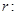100 is divisible by 5.

The component statementsandare false whereas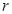is true.